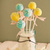## pandas 转

（2）十分钟入门Pandas： 10 Minutes to pandas

## 关键缩写和包导入

df：任意的Pandas DataFrame对象

import pandas as pd

## 导入数据

• pd.read_csv(filename)：从CSV文件导入数据
• pd.read_table(filename)：从限定分隔符的文本文件导入数据
• pd.read_excel(filename)：从Excel文件导入数据
• pd.read_sql(query, connection_object)：从SQL表/库导入数据
• pd.read_json(json_string)：从JSON格式的字符串导入数据
• pd.read_html(url)：解析URL、字符串或者HTML文件，抽取其中的tables表格
• pd.read_clipboard()：从你的粘贴板获取内容，并传给read_table()
• pd.DataFrame(dict)：从字典对象导入数据，Key是列名，Value是数据

## 导出数据

• df.to_csv(filename)：导出数据到CSV文件
• df.to_excel(filename)：导出数据到Excel文件
• df.to_sql(table_name, connection_object)：导出数据到SQL表
• df.to_json(filename)：以Json格式导出数据到文本文件

## 创建测试对象

• pd.DataFrame(np.random.rand(20,5))：创建20行5列的随机数组成的DataFrame对象
• pd.Series(my_list)：从可迭代对象my_list创建一个Series对象
• df.index = pd.date_range('1900/1/30', periods=df.shape)：增加一个日期索引

## 查看、检查数据

• df.head(n)：查看DataFrame对象的前n行
• df.tail(n)：查看DataFrame对象的最后n行
• df.shape()：查看行数和列数
• http:// df.info() ：查看索引、数据类型和内存信息
• df.describe()：查看数值型列的汇总统计
• s.value_counts(dropna=False)：查看Series对象的唯一值和计数
• df.apply(pd.Series.value_counts)：查看DataFrame对象中每一列的唯一值和计数

## 数据选取

• df[col]：根据列名，并以Series的形式返回列
• df[[col1, col2]]：以DataFrame形式返回多列
• s.iloc：按位置选取数据
• s.loc['index_one']：按索引选取数据
• df.iloc[0,:]：返回第一行
• df.iloc[0,0]：返回第一列的第一个元素

## 数据清理

• df.columns = ['a','b','c']：重命名列名
• pd.isnull()：检查DataFrame对象中的空值，并返回一个Boolean数组
• pd.notnull()：检查DataFrame对象中的非空值，并返回一个Boolean数组
• df.dropna()：删除所有包含空值的行
• df.dropna(axis=1)：删除所有包含空值的列
• df.dropna(axis=1,thresh=n)：删除所有小于n个非空值的行
• df.fillna(x)：用x替换DataFrame对象中所有的空值
• s.astype(float)：将Series中的数据类型更改为float类型
• s.replace(1,'one')：用‘one’代替所有等于1的值
• s.replace([1,3],['one','three'])：用'one'代替1，用'three'代替3
• df.rename(columns=lambda x: x + 1)：批量更改列名
• df.rename(columns={'old_name': 'new_ name'})：选择性更改列名
• df.set_index('column_one')：更改索引列
• df.rename(index=lambda x: x + 1)：批量重命名索引

## 数据处理：Filter、Sort和GroupBy

• df[df[col] > 0.5]：选择col列的值大于0.5的行
• df.sort_values(col1)：按照列col1排序数据，默认升序排列
• df.sort_values(col2, ascending=False)：按照列col1降序排列数据
• df.sort_values([col1,col2], ascending=[True,False])：先按列col1升序排列，后按col2降序排列数据
• df.groupby(col)：返回一个按列col进行分组的Groupby对象
• df.groupby([col1,col2])：返回一个按多列进行分组的Groupby对象
• df.groupby(col1)[col2]：返回按列col1进行分组后，列col2的均值
• df.pivot_table(index=col1, values=[col2,col3], aggfunc=max)：创建一个按列col1进行分组，并计算col2和col3的最大值的数据透视表
• df.groupby(col1).agg(np.mean)：返回按列col1分组的所有列的均值
• data.apply(np.mean)：对DataFrame中的每一列应用函数np.mean
• data.apply(np.max,axis=1)：对DataFrame中的每一行应用函数np.max

## 数据合并

• df1.append(df2)：将df2中的行添加到df1的尾部
• df.concat([df1, df2],axis=1)：将df2中的列添加到df1的尾部
• df1.join(df2,on=col1,how='inner')：对df1的列和df2的列执行SQL形式的join

## 数据统计

• df.describe()：查看数据值列的汇总统计
• df.mean()：返回所有列的均值
• df.corr()：返回列与列之间的相关系数
• df.count()：返回每一列中的非空值的个数
• df.max()：返回每一列的最大值
• df.min()：返回每一列的最小值
• df.median()：返回每一列的中位数
• df.std()：返回每一列的标准差

### 评论(1)df.rename(columns=lambda x: x + 1)：批量更改列名
df.rename(columns={'old_name': 'new_ name'})：选择性更改列名
df.set_index('column_one')：更改索引列
df.rename(index=lambda x: x + 1)：批量重命名索引

list=[xxx,xxx,xxx,xxx,......]
csv=read_csv(file,header=0,names=list)

csv=read_csv(file,header=0)
csv.columns=names

Pandas初学者代码优化指南

dev_csdn
2017/11/21
0
0
Koalas：让 pandas 轻松切换 Apache Spark

4 月 24 日，Databricks 在 Spark + AI 峰会上开源了一个新产品 Koalas，它增强了 PySpark 的 DataFrame API，使其与 pandas 兼容。 Python 数据科学在过去几年中爆炸式增长， pandas 已成为...

05/06
0
0
Koalas: 让 pandas 开发者轻松过渡到 Apache Spark

Spark
04/29
0
0
Python数据分析(一) 关于pandas和numpy

2018/04/14
0
0

2018/04/22
0
0

OSChina 周六乱弹 —— 早上儿子问我他是怎么来的

Osc乱弹歌单（2019）请戳（这里） 【今日歌曲】 @凉小生 ：#今日歌曲推荐# 少点戾气，愿你和这个世界温柔以待。中岛美嘉的单曲《僕が死のうと思ったのは (曾经我也想过一了百了)》 《僕が死の...

1K
12
Excption与Error包结构，OOM 你遇到过哪些情况，SOF 你遇到过哪些情况

Throwable 是 Java 中所有错误与异常的超类，Throwable 包含两个子类，Error 与 Exception 。用于指示发生了异常情况。 Java 抛出的 Throwable 可以分成三种类型。 被检查异常（checked Exc...

Garphy

30
0

FAT_mt

28
0

44
0
tabel 中含有复选框的列 数据理解

1、el-ui中实现某一列为复选框 实现多选非常简单: 手动添加一个el-table-column，设type属性为selction即可； 2、@selection-change事件：选项发生勾选状态变化时触发该事件 <el-table @sel...

everthing

17
0